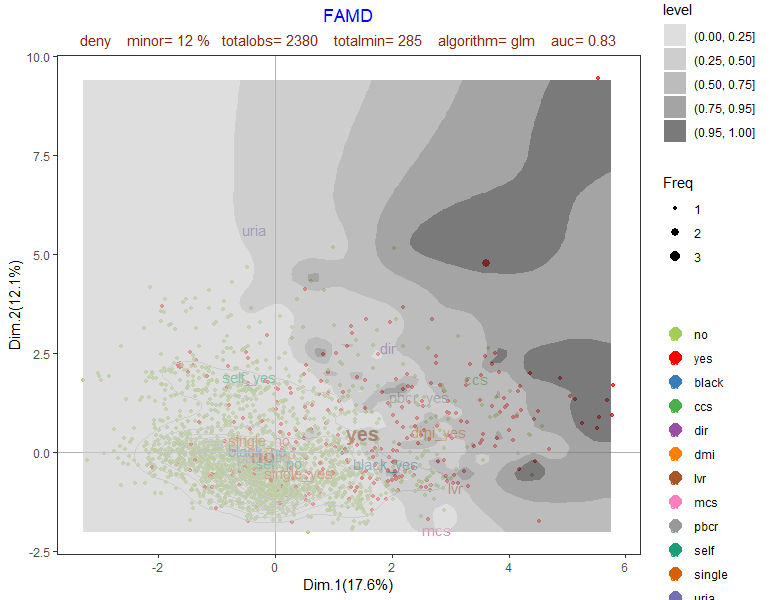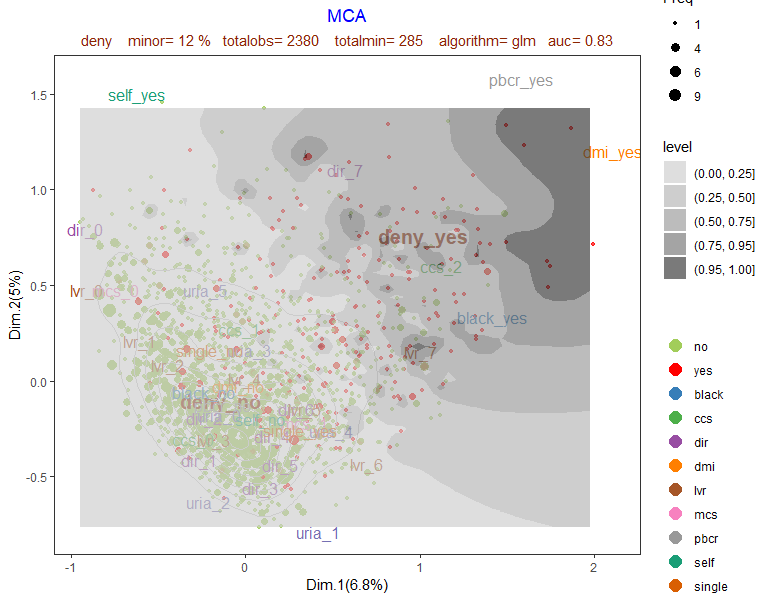# Basic example with FAMD

Factorial Analysis for Mixed Data, applied to the space of input variables, is used to represent points in two dimensions. Points are colored based on dependent classification variable in order to reveal the discriminant power of input variables. 6 plots are generated. Contour plots are built from interpolation on a grid, departing from predicted probabilities for actual data. Some plots project interval input variable and categories of class variables. Other plots show fitted probabilities and absolute difference of fitted probability from real value of dependent variable coded to {0,1}. Logistic regression (glm) is used as default predicting algorithm. In this example a previous stepwise variable selection is performed (selec=1). By default selec=0. Order of plots shown is result[], result[], etc.

``````library(visualpred)
dataf<-breastwisconsin1
listconti=c( "clump_thickness","uniformity_of_cell_shape",
"bland_chromatin", "normal_nucleoli", "mitosis")
listclass=c("")
vardep="classes"
result<-famdcontour(dataf=dataf,listconti,listclass,vardep,title="FAMD Plots",selec=1)
``````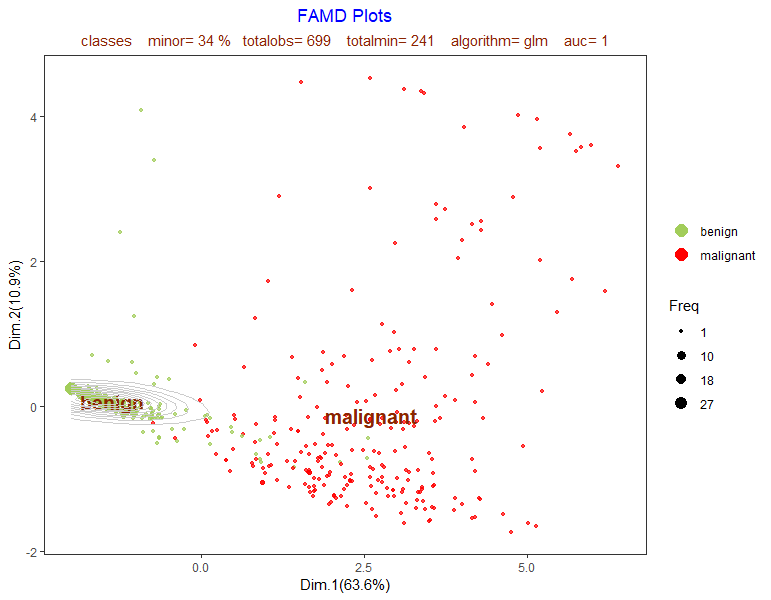The plot above shows observations projected on first chosen two FAMD dimensions. The plot reveals how input variables can discriminate between categories of the dependent class variable.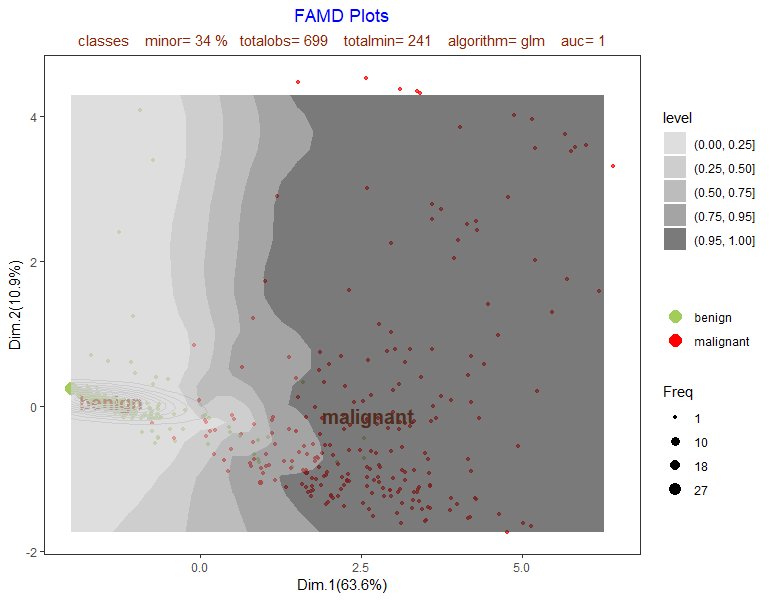This plot above shows contour plot based on glm sample predicted probabilities .

\$graph3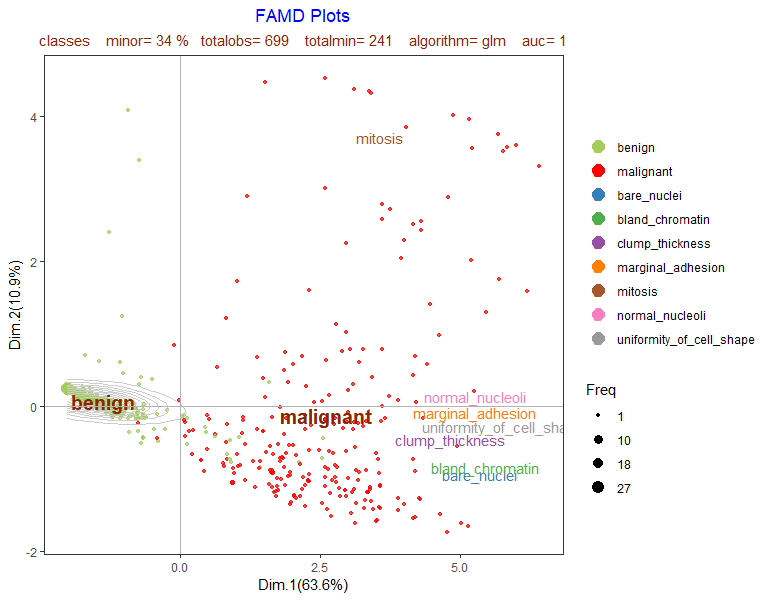This plot above is first plot adding interval input variables coordinates.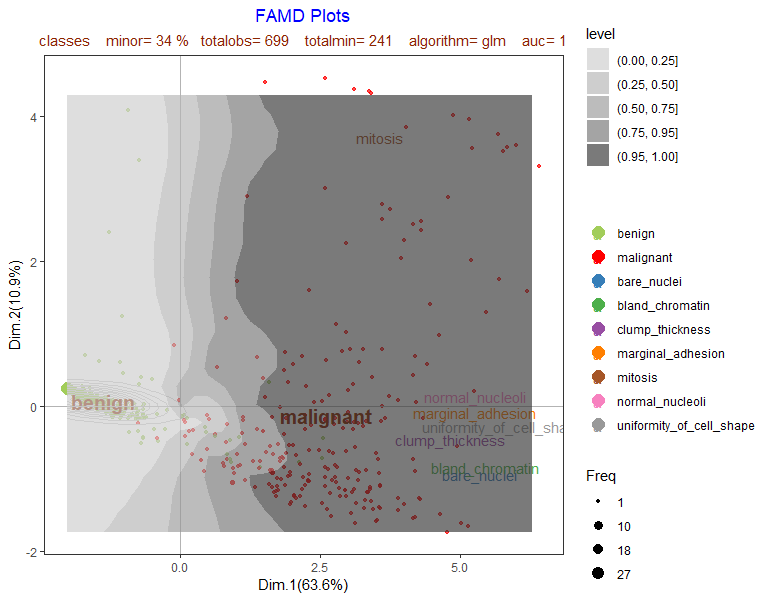This plot above is second plot adding interval input variables coordinates.

\$graph5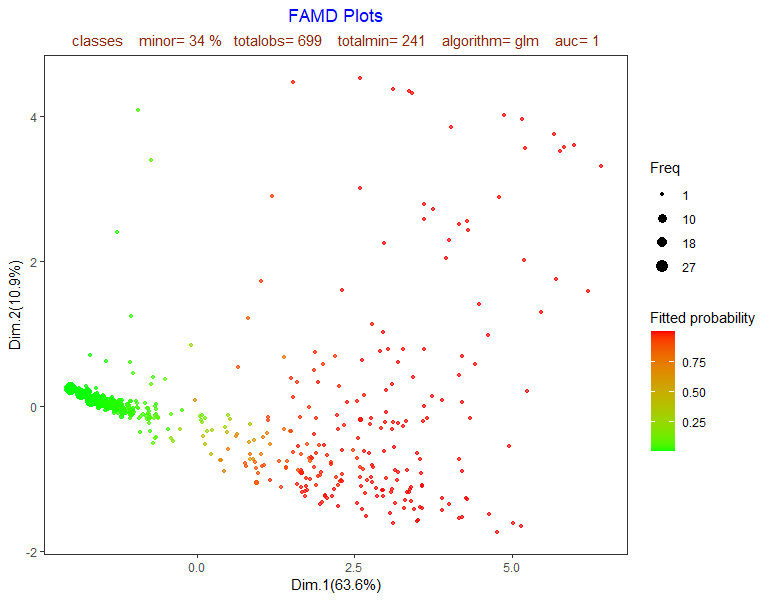This plot above shows fitted probabilities in color scale.

\$graph6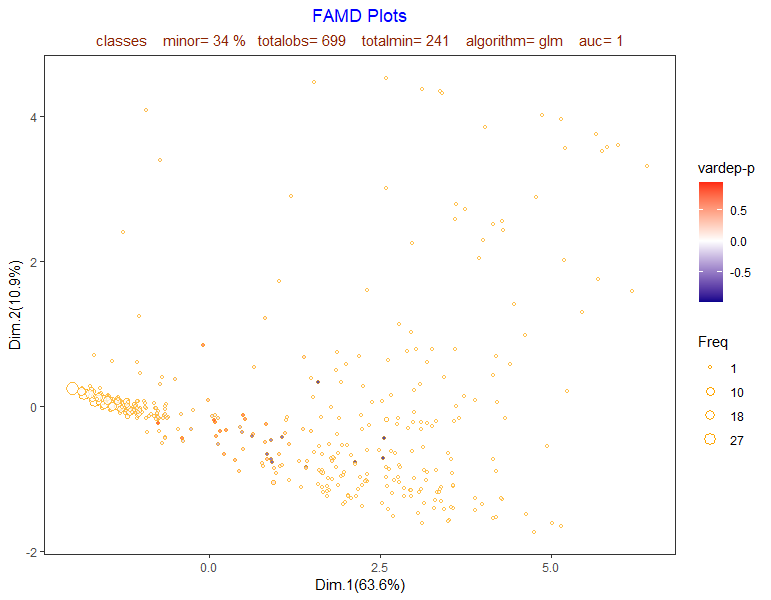This plot above shows difference between dependent variable (categorized as 0,1) and predicted probabilities.

# Differences between mcacontour and famdcontour

In the next example a dataset with only interval input variables is used. Function mcacontour categorizes the input interval variables whereas famdcontour does not. It seems more appropriate to use famd, but interesting predictive clues are obtained in the mca variables plot.

``````library(visualpred)
dataf<-pima
listconti<-c("pregnant", "glucose", "pressure", "triceps", "insulin", "bodymass",
"pedigree", "age")
listclass<-c("")
vardep<-"diabetes"

resultfamd<-famdcontour(dataf=pima,listconti,listclass,vardep,title="FAMD",selec=1,
alpha1=0.7,alpha2=0.7,proba="",modelo="glm")
resultmca<-mcacontour(dataf=pima,listconti,listclass,vardep,title="MCA",proba="",
modelo="glm",selec=1,alpha1=0.7,alpha2=0.7)
``````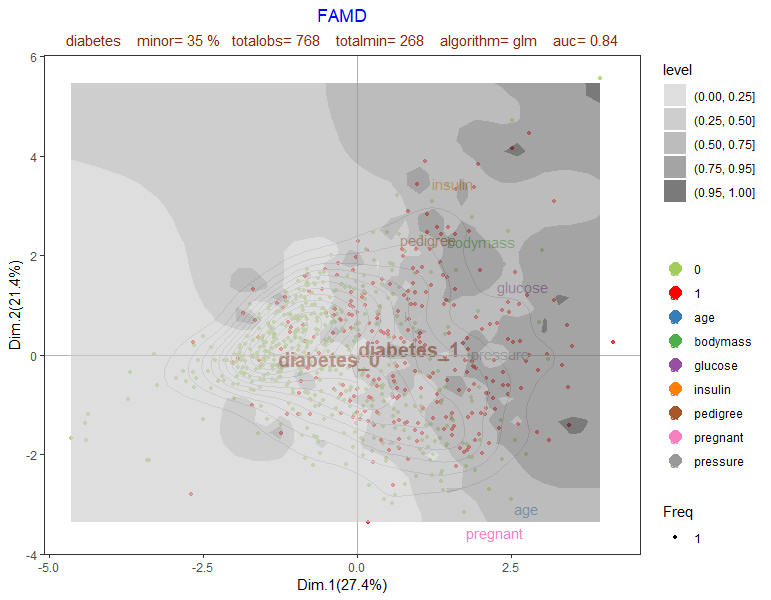Since there are only interval variables famd is able to represent a better point projections, where predictive contour curves are natural and clear . The projection of variable labels show their association with dependent class variable.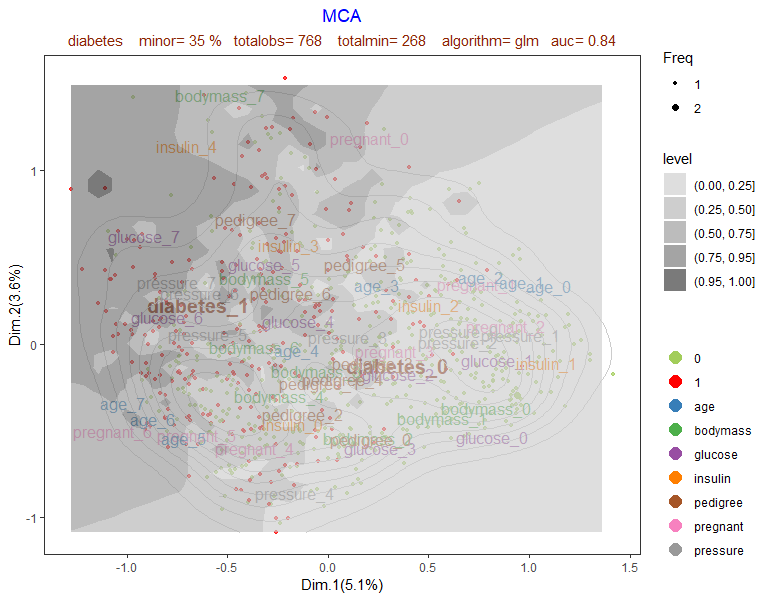Mca plot seems less informative about general contour model fit, but as interval variables are categorized (by default in 8 bins), it shows other interesting associations: pregnant variable=0 could be treated as a dummy category in itself. Also bodymass shows a non linear relationship for classification of the dependent variable. Insulin_0 (lower value of insulin bins) also seems to be a category of interest. None of these clues could be found in previous famd plot.

# Dataset with both input class and interval variables

``````library(visualpred)
dataf<-na.omit(Hmda)
listconti<-c("dir", "hir", "lvr", "ccs", "mcs", "uria")
listclass<-c("pbcr", "dmi", "self", "single", "condominium", "black")
vardep<-c("deny")
resultfamd<-famdcontour(dataf=Hmda,listconti,listclass,vardep,title="FAMD",selec=1,
alpha1=0.7,alpha2=0.7,proba="",modelo="glm")
resultmca<-mcacontour(dataf=Hmda,listconti,listclass,vardep,title="MCA",proba="",
modelo="glm",selec=1,alpha1=0.7,alpha2=0.7)
``````